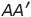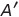Select Page

# Solutions for MCQ CBSE 12 Science Maths Differential Equations in Hindi

Solutions for MCQ CBSE 12 Science Maths Differential Equations in Hindi to enable students to get Solutions in a narrative video format for the specific question.

Expert Teacher provides Solutions for MCQ CBSE 12 Science Maths Differential Equations through Video Solutions in Hindi language. This video solution will be useful for students to understand how to write an answer in exam in order to score more marks. This teacher uses a narrative style for a question from Differential Equations not only to explain the proper method of answering question, but deriving right answer too.

Please find the question below and view the Solution in a narrative video format.

Question:

Solution Video in Hindi:

You can select video Solutions from other languages also. Please check Solutions in ( English )

## Similar Questions from CBSE, 12th Science, Maths, Differential Equations

Question 1 : Write the differential equation representing the family of curves y = mx, where m is an arbitrary constant. (View Answer Video)

Question 2 : Find the general solution of differential equation(View Answer Video)

Question 3 : Solve the differential equation(View Answer Video)

Question 4 : Write the degree of the differential equation :(View Answer Video)

Question 5 : Write the sum of the order and degree of the differential equation(View Answer Video)

### Determinant

Question 1 : The solution of the following system of equation is 2x + 3y = 5, 5x – 2y = 3. (View Answer Video)

Question 2 : Let A be a non-singular square matrix of order 3 * 3. Then | adj A | is equal to: (View Answer Video)

Question 3 : The following system of equations has x + 3y + 3z = 2, x + 4y + 3z = 1, x + 3y + 4z = 2,

Question 4 : Iffind |A|. (View Answer Video)

Question 5 : Evaluate. (View Answer Video)

### Matrices

Question 1 : If, write, whereis the transpose of A? (View Answer Video)

Question 2 : If, then find "a". (View Answer Video)

Question 3 : Find the value of z,  from the equation:. (View Answer Video)

Question 4 : If, write the value of"x". (View Answer Video)

Question 5 : Given,, find the value of y. (View Answer Video)

### Continuity and Differentiability

Question 1 :  Find the second order derivative of the function. (View Answer Video)

Question 2 : Differentiate the functionwith respect to x. (View Answer Video)

Question 3 : Differentiate w.r.t.x the function. (View Answer Video)

Question 4 : Findfor the function. (View Answer Video)

Question 5 : If x and y are connected parametrically by the equation, without eliminating the parameter, find. (View Answer Video)